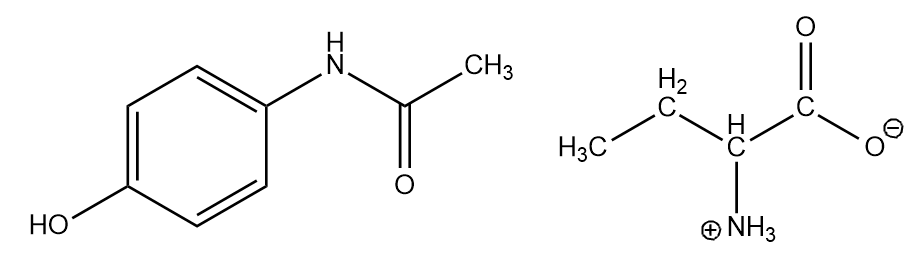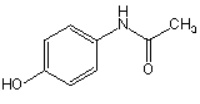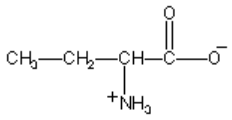# Problem: How many kinds of chemically non-equivalent hydrogens are there in each of the following compounds?a) Tylenol (analgesic and antipyretic) b)

###### FREE Expert Solution

For this problem, we need to determine how many kinds of chemically non-equivalent Hs in each of the ff. compounds• Recall that for 1H-NMR analysis, there are as many signals on each spectrum as there are unique, non-equivalent protons
• In this case, let's assume that Hs bound to the same C is equivalent
• Take note that symmetry will reduce the total number of signals
92% (246 ratings)###### Problem Details

How many kinds of chemically non-equivalent hydrogens are there in each of the following compounds?

a) Tylenol (analgesic and antipyretic)b)